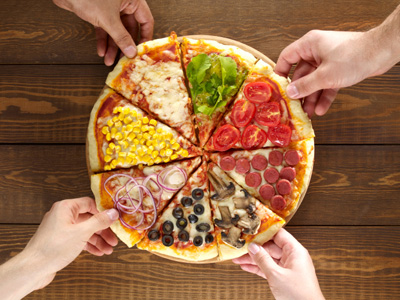If 8 slices of pizza are shared between 4 people they get 2 slices each.

# Year 2 Calculation - Division (Mental Methods)

This quiz addresses the requirements of the National Curriculum KS1 Maths and Numeracy for children aged 6 and 7 in year 2. Specifically this quiz is aimed at the section dealing with using mental methods for division.

Understanding the process of division can be quite a tricky challenge for some children. Children may be initially introduced to the idea of 'sharing' equally before they are shown how to use mental methods to solve problems. This starts when they begin to understand the division facts related to the multiplication facts they are learning (for example, if they know that 2 x 5 = 10, than they could recognise that 10 ÷ 5 = 2). They will learn that division is, in fact, the same as repeated subtraction and also understand that whilst the multiplication of two numbers can be done in any order (commutative), the division of one number by another cannot.

This quiz will help your child to solve division problems using the mental methods they have learnt.

Question 1
If I share 6 sweets equally into 3 bags, how many will be in each bag?
6 x 2 = 3
3 ÷ 2 = 6
6 ÷ 3 = 2
2 ÷ 3 = 6
If we divide the 6 sweets equally, there will be 2 sweets in each bag
Question 2
If you know that 5 x 2 = 10 and 2 x 5 = 10, what other facts do you know?
10 x 5 = 2 and 2 ÷ 5 = 10
2 ÷ 5 = 10 and 5 ÷ 2 = 10
2 x 10 = 5 and 5 x 10 = 2
10 ÷ 5 = 2 and 10 ÷ 2 = 5
The division facts always have the largest number at the beginning
Question 3
5 x 2 = 10
20 x 5 = 100
5 x 20 = 4
5 x 4 = 20
Knowing the multiplication tables well will help you to work out the division facts
Question 4
What is 12 divided by 6?
12 ÷ 6 = 2
12 ÷ 6 = 18
12 ÷ 6 = 72
12 ÷ 6 = 4
We could also say that 12 ÷ 2 = 6
Question 5
Which two division calculations could apply to the number 8?
8 ÷ 4 = 2 and 8 ÷ 2 = 4
6 ÷ 3 = 2 and 6 ÷ 2 = 3
10 ÷ 2 = 5 and 10 ÷ 5 = 2
12 ÷ 6 = 2 and 12 ÷ 2 = 6
If we divide 8 tomatoes into 2 groups, there would be 4 in each group. If we divided them into 4 groups, there would be 2 in each group
Question 6
Division is the same as...
finding the total
repeated subtraction
multiplying
adding up over and over again
6 ÷ 3 gives the same answer as taking 3 away from 6 until none remain
Question 7
4 friends share 8 slices of pizza. How many slices will they each get?
2, because 8 ÷ 4 = 2
4, because 8 ÷ 2 = 4
8, because 8 ÷ 1 = 8
1, because 8 ÷ 8 = 1
If you know that 4 x 2 = 8, then you could work out that 8 ÷ 4 = 2
Question 8
If I divided 9 photographs into piles of 3, how many piles could I make?
9, because 9 ÷ 1 = 9
3, because 9 ÷ 3 = 3
1, because 9 ÷ 9 = 1
27, because 9 x 3 = 27
If you know that 3 x 3 = 9, it could help you work out that 9 ÷ 3 = 3
Question 9
I know that 6 x 3 = 18. Which fact is the odd one out?
3 x 6 = 18
18 ÷ 6 = 3
18 ÷ 3 = 6
18 ÷ 9 = 2
The division and multiplication facts form a 'family' of four - two division and two multiplication
Question 10
Which division fact is incorrect?
14 ÷ 7 = 2
16 ÷ 2 = 8
20 ÷ 10 = 2
10 ÷ 3 = 7
The others are all related to the 2 times table